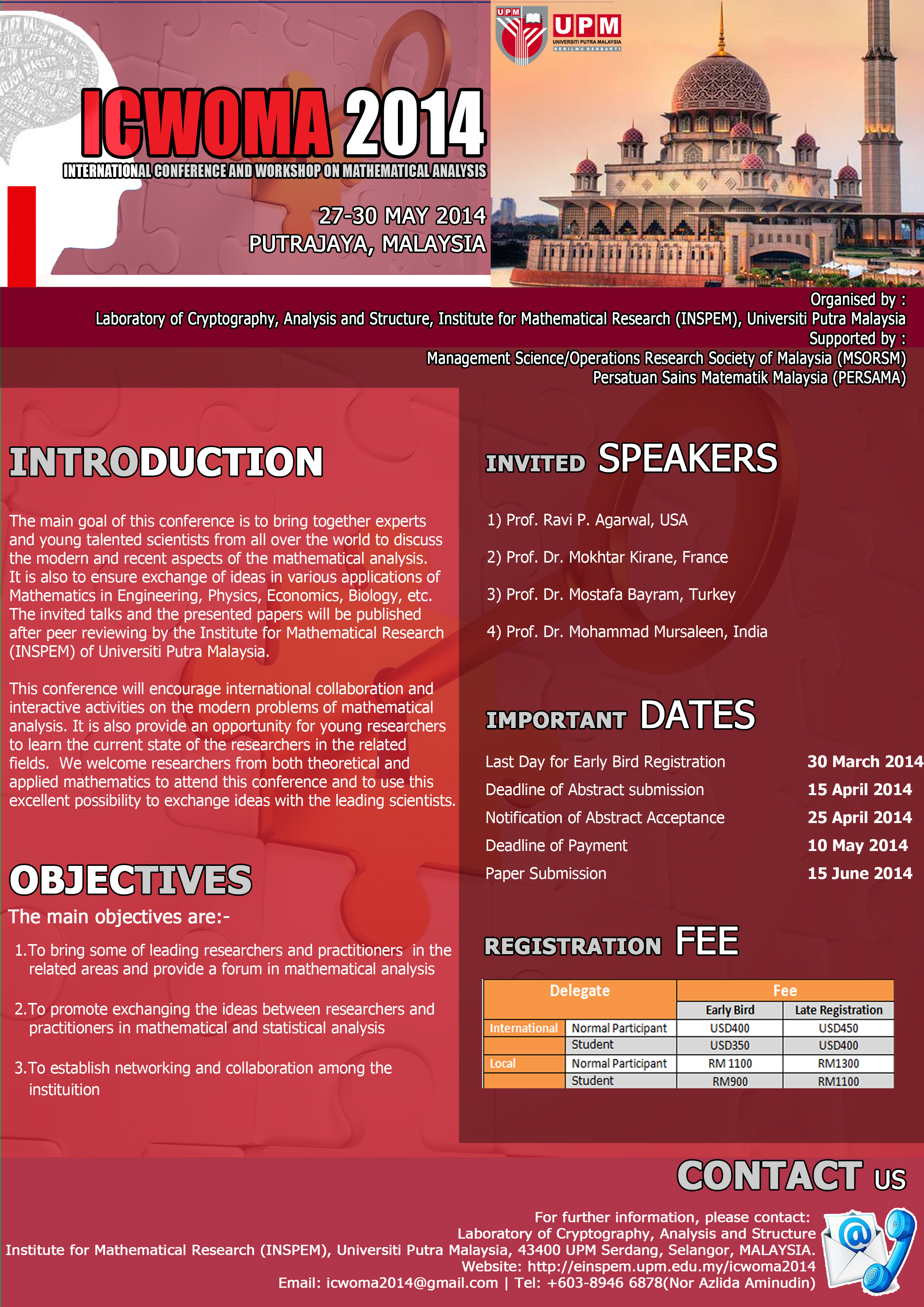Introduction

Optimization can be broadly defined as the process of selecting a set of best parameters from some available alternatives subjecting to stated constraints and meeting specific objectives. Generally, the optimization process involves maximizing or minimizing a real mathematic function by systematically choosing input values from within an allowed set and computing the value of the function. Due to its nature, optimization has found broad usage in engineering, economics and science.

This workshop is intended as a practical introduction to solving optimization problems using traditional optimization techniques such as unconstrained optimization, nonlinear programming with equality and inequality constraints, and convex programming.

Objectives
1. Practical introduction to solving optimization problems using traditional optimization techniques such as unconstrained optimization, nonlinear programming with equality and inequality constraints, and convex programming.
2. To understand the application of the main methods of optimization to real world problems. Different examples are offered to help the students understand solution strategy.
3. Solution strategy of Convex programming problems.
What You Will Learn
1. To solve unconstrained optimization methods.
2. To solve constrained optimization methods with equality and inequality constraints. Application of 1st and 2nd order necessary and sufficient conditions of optimality.
3. Solution strategy of Convex programming problems.
Who Should Attend

MSc and PhD students of all faculties of UPM and other universities, and researchers who wish to learn about solving optimization problems. No background in optimization theory is, however, assumed.

Announcements:

Registration Dateline:
23 April 2014

Payment Dateline:
23 April 2014